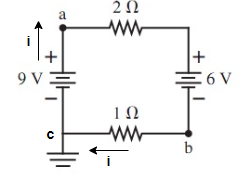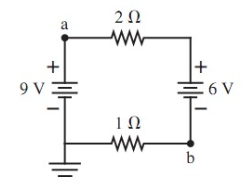# Problem: In the following figure, what is the value of the potential at points a and b? (Figure 1) Express your answer using two significant figures.

###### FREE Expert Solution
100% (104 ratings)
###### FREE Expert Solution

Kirchhoff's loop rule:

$\overline{){\mathbf{\Sigma }}{\mathbf{V}}{\mathbf{=}}{\mathbf{0}}}$Starting from point a

$\begin{array}{rcl}\mathbf{-}\mathbf{i}\mathbf{2}\mathbf{\Omega }\mathbf{-}\mathbf{6}\mathbf{V}\mathbf{-}\mathbf{i}\mathbf{1}\mathbf{\Omega }\mathbf{+}\mathbf{9}\mathbf{V}& \mathbf{=}& \mathbf{0}\\ \mathbf{-}\mathbf{i}\mathbf{3}& \mathbf{=}& \mathbf{-}\mathbf{3}\mathbf{V}\end{array}$

i = 1A

100% (104 ratings)###### Problem Details

In the following figure, what is the value of the potential at points a and b? (Figure 1) Express your answer using two significant figures.